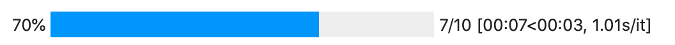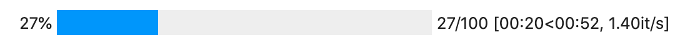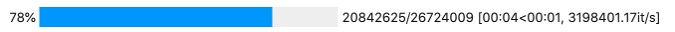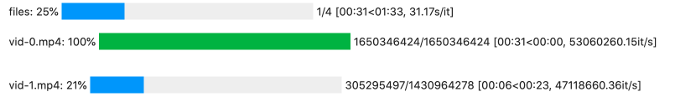# Show progress in your Python apps with tqdm

## If your program takes a while to show results, avoid frustrating users by showing the progress it's making.Image by :
Opensource.com

## Subscribe now

Get the highlights in your inbox every week.

The Semitic root q-d-m in Aramaic, Hebrew, and Arabic is usually associated with moving forward or making progress. The Arabic word taqaddum (تقدّم) means "progress." Progress is important. As every feel-good movie will tell you, the journey is as important as the destination.

Most programs have a clear goal, a desired end state. Sometimes, calculating that end state can take a long time. While computers don't care, not having feelings, people do. Humans are not happy sitting around waiting without any visible sign of progress. Doubt creeps in. Has the program crashed? Is the disk thrashing? Did the operating system allocate all its computing resources to other tasks?

Like justice, progress must be seen, not merely done. The tqdm Python library helps make progress explicit.

The tqdm module works with the console, but it also has special support for one of my favorite environments: Jupyter. To use tqdm in Jupyter, you need to import the `notebook` submodule and have ipywidgets installed. The `notebook` submodule is interface-compatible with tqdm.

This means you can do some import-time shenanigans to import the correct module while keeping tqdm usage the same. The trick is to check if the `__main__` module has the global variable `get_ipython`. While this is a heuristic, it is a reasonably accurate one:

```import sys
if hasattr(sys.modules["__main__"], "get_ipython"):
from tqdm import notebook as tqdm
else:
import tqdm```

The simplest case is when something needs to run for a certain number of iterations (known in advance), and each of those iterations takes about the same amount of time. For example, there is an algorithm to calculate the square root of any number by starting with 1 as a guess and then calculating an improved guess:

```def improve_guess(rt, n):
return (rt + n/rt) / 2```

A small number of improvements gets you pretty close. For example, you can calculate the square root of two:

```guess = 1
target = 2
for i in tqdm.trange(10):
guess = improve_guess(guess, target)```

## output_8_0.pngIt's correct to 10 decimal places!

``round(2 - guess*guess, 10)``
``0.0``

A slightly more complicated example is when the number of elements is known, and processing each element takes a similar amount of time. As an example, you can calculate the product of some numbers. For that, you'll want some random numbers:

```import random
numbers = [random.uniform(0, 2.8) for i in range(100)]
numbers[:5]```
```[2.6575636572230916,
0.1286674965830302,
1.0634250104041332,
1.1760969844376505,
0.45192978568125486]```

Now that the numbers are in, it's time to multiply them. The easiest way to use tqdm is by wrapping a Python iterable. The values will be the same, but tqdm will also display a progress bar:

```result = 1
for num in tqdm.tqdm(numbers):
result *= num
result```
``2.4081854901728303``

## output_15_0.pngHowever, not all things are predictable. One of the least predictable things is network speed. When you download a big file, the only way to measure progress is to explicitly check how much has been downloaded:

```url = "https://www.python.org/ftp/python/3.9.0/Python-3.9.0.tgz"
import httpx
with httpx.stream("GET", url) as response:
with tqdm.tqdm(total=total) as progress:
for chunk in response.iter_bytes():
progress.update(len(chunk))```

## output_18_0.pngSometimes, it makes sense to "nest" progress bars. For example, if you are downloading a directory, you'll want a progress bar tracking the files and a progress bar per file.

```files = [f"vid-{i}.mp4" for i in range(4)]
for fname in tqdm.tqdm(files, desc="files"):
total = random.randrange(10**9, 2 * 10**9)
with tqdm.tqdm(total=total, desc=fname) as progress:
current = 0
while current < total:
chunk_size = min(random.randrange(10**3, 10**5), total - current)
current += chunk_size
if random.uniform(0, 1) < 0.01:
time.sleep(0.1)
progress.update(chunk_size)```

## output_21_0.png